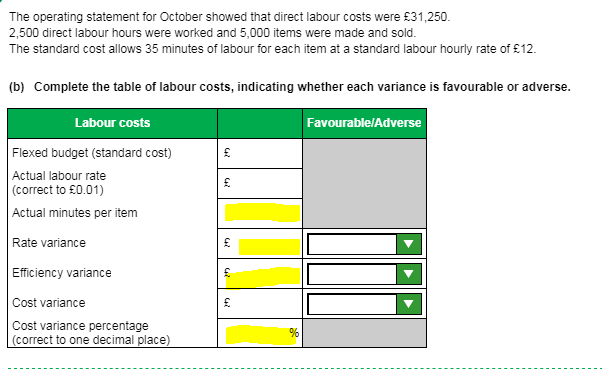# Task 6b practice assessment 2

Hi,

I know how to work out most of this but i'm stuck on actual mins per item, rate variance, efficiency variance and variance %. i have my exam next week and i'm panicking i feel like I've forgot everything.

Thanks
Sophie## Comments

• Actual costs are £31250, actual hours are 2500 and actual items made are 5000.

I'll just work my way down the question so it flows.
Standard cost is standard minutes of 35 mins per item converted into hours, so ((35/60)x actual items 5000) then multiply by standard hourly rate of £12 to get 35000 as your flexed budget. So, your variances for rate and efficiency should tie back to actual cost of £31250 - £35000, so we are looking for 3750 F all together.

actual minutes per item, so its actual hours 2500 / actual items 5000 = 0.5 HOURS per item, but you need to know minutes so times 0.5 x 60 = 30 minutes.
RATE variance - how much we shouldve spent on labour. We shouldve spent standard £12 per hour. 12 x actual hours of 2500 = 30,000 but we actually spent £31250 as per the question so variance of £1250 ADV

EFFICIENCY- how many hours we shouldve spent times by standard rate.
standard is 35 mins. So 35/60 x actual items = 2916.666667 hours. Actual hours were 2500. 2916.666667-2500 = 416.6666667 HOURS fav. Times the hours variance by standard hourly rate of £12 and you get £5000 FAV

£5000 F and £1250 A gives you £3750 FAV all together as per original.

cost variance percentage is simply £3750 / standard flexed budget of £35000 x100 to get % = 10.7

Hope this makes sense!

• > @Kdriver1 said:
> Actual costs are £31250, actual hours are 2500 and actual items made are 5000.
>
> I'll just work my way down the question so it flows.
> Standard cost is standard minutes of 35 mins per item converted into hours, so ((35/60)x actual items 5000) then multiply by standard hourly rate of £12 to get 35000 as your flexed budget. So, your variances for rate and efficiency should tie back to actual cost of £31250 - £35000, so we are looking for 3750 F all together.
>
> actual minutes per item, so its actual hours 2500 / actual items 5000 = 0.5 HOURS per item, but you need to know minutes so times 0.5 x 60 = 30 minutes.
> RATE variance - how much we shouldve spent on labour. We shouldve spent standard £12 per hour. 12 x actual hours of 2500 = 30,000 but we actually spent £31250 as per the question so variance of £1250 ADV
>
> EFFICIENCY- how many hours we shouldve spent times by standard rate.
> standard is 35 mins. So 35/60 x actual items = 2916.666667 hours. Actual hours were 2500. 2916.666667-2500 = 416.6666667 HOURS fav. Times the hours variance by standard hourly rate of £12 and you get £5000 FAV
>
> £5000 F and £1250 A gives you £3750 FAV all together as per original.
>
> cost variance percentage is simply £3750 / standard flexed budget of £35000 x100 to get % = 10.7
>
> Hope this makes sense!

@Kdriver1 thank you so much for taking the time to write all this for me
Sign In or Register to comment.